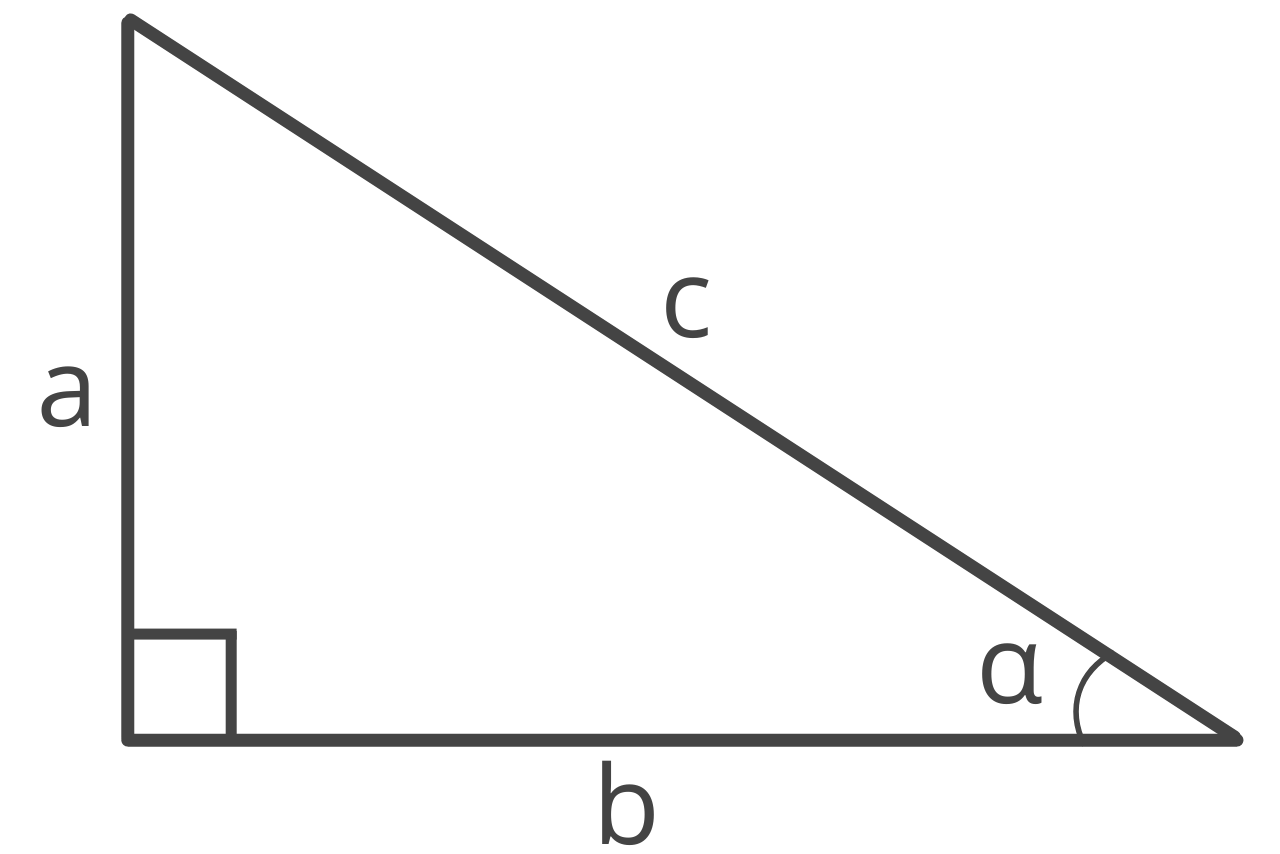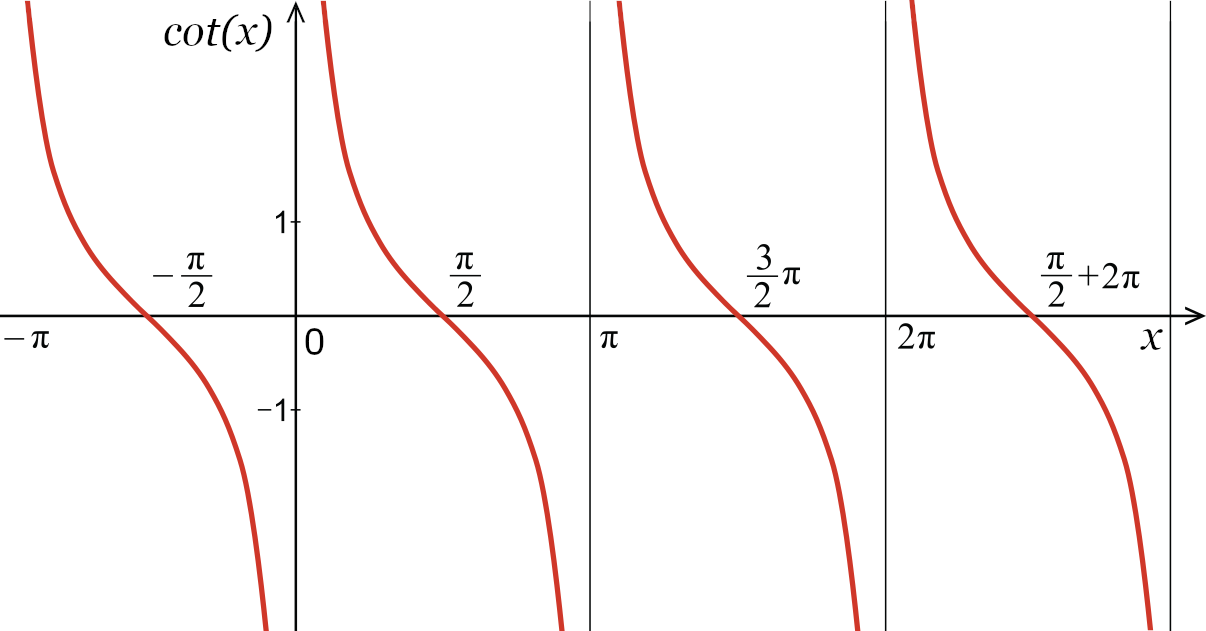# Cotangent Calculator – Calculate cot(x)

Find the cotangent of an angle using the cot calculator below. Start by entering the angle in degrees or radians.

## How to Find the Cotangent of an Angle

In a right triangle, the cotangent of angle α, or cot(α), is the ratio between the adjacent side of an angle and the opposite side.Cotangent is a trigonometric function abbreviated cot. Use the formula below to calculate the cotangent of an angle.

### Cotangent Formula

The cotangent formula is:

cot(α) = adjacent / opposite = b / a

Thus, the cotangent of angle α in a right triangle is equal to the length of the adjacent side b divided by the opposite side a.

To find the ratio of cotangent, simply enter the length of the adjacent and opposite sides, then simplify.This formula might look very similar to the formula to calculate tangent. That’s because cotangent is the reciprocal of tangent.

For example, let’s calculate the cotangent of angle α in a triangle with the length of the adjacent side equal to 8 and the opposite side equal to 4.

cot(α) = 8 / 4 = 2 / 1

## Cotangent vs. Arctan

Cotangent should not be confused with arctan, which is the inverse of the tangent function. The difference being that cotangent is equal to 1/tan(x), while arctan is the inverse of the tangent function.

cot(x) = 1 / tan(x) = [tan(x)]-1

Whereas,

arctan(y) = x or tan-1(y) = x when y = tan(x)

## Cotangent Graph

If you graph the cotangent function for every possible angle, it forms multiple decreasing curves with an asymptote at the angle 0 and repeating every π radians, or 180°. See values in the table below.One important property to note in the graph above is that the cotangent of an angle is never equal to 0 or an even multiple of π radians, or 180°. The x-intercepts occur in each period and are separated by a distance of one period.

The cotangent function continues indefinitely and has a period of π.

## Cotangent Table

The table below shows common angles and the cot value for each of them.

-√3

Table showing common angles and cot values for each.
0 undefined
15° π / 12 2 + √3
30° π / 6 √3
45° π / 4 1
60° π / 3 1 / √3 = √3 / 3
75° / 12 2 – √3
90° π / 2 0
105° / 12 -2 + √3
120° / 3 1 / √3 = –√3 / 3
135° / 4 -1
150° / 6 -√3
165° 11π / 12 -2 – √3
180° π undefined
195° 13π / 12 2 + √3
210° / 6 √3
225° / 4 1
240° / 3 1 / √3 = √3 / 3
255° 17π / 12 2 – √3
270° / 2 0
285° 19π / 12 -2 + √3
300° / 3 1 / √3 = –√3 / 3
315° / 4 -1
330° 11π / 6
345° 23π / 12 -2 – √3
360° undefined

You might also be interested in our secant and cosecant calculators.

### Is cotangent the inverse of tangent?

Tangent and cotangent are not inverse functions. The inverse of tangent is the arctangent, while the inverse of cotangent is the arccotangent function.

### Do you use degrees or radians with cotangent?

Although you can use either degrees or radians with cotangent, radians are the most common unit of measurement. This is due to the simplicity when calculating formulas that involve trigonometric functions in radians.

### Can you find cotangent without a calculator?

To find the cotangent of an angle without a calculator, you need to know the unit circle and the trigonometric ratios. The cotangent of an angle x is the ratio of the adjacent side to the opposite side of a right triangle that contains the angle x.

Therefore, to find the cotangent of an angle x, you need to first identify the adjacent and opposite sides of a right triangle that contains the angle x, and then take the ratio of these two sides.

### How do you find cotangent on a calculator?

To find the cotangent of an angle with a calculator, begin by entering the angle in degrees or radians (depending on what mode you calculator is in). Press the cot button on the calculator to find the cotangent of the angle.

If your calculator doesn’t have a cot or cotan button, you can enter the tangent of the angle and then press 1/x or the reciproc button to find cotangent.